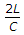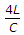# Civil Engineering - Hydraulics

### Exercise :: Hydraulics - Section 1

6.

The total pressure force on a plane area is equal to the area multiplied by the intensity of pressure at its centroid, if

 A. area is horizontal B. area is vertical C. area is inclined D. all the above.

Explanation:

No answer description available for this question. Let us discuss.

7.

If the volume of a liquid weighing 3000 kg is 4 cubic metres, 0.75 is its

 A. specific weight B. specific mass C. specific gravity D. none of these.

Explanation:

No answer description available for this question. Let us discuss.

8.

Bernoulli's equation assumes that

 A. fluid is non-viscous B. fluid is homogeneous C. flow is steady D. flow is along the stream line E. all the above.

Explanation:

No answer description available for this question. Let us discuss.

9.

The time required to close a valve gradually is (where L is the length of pipe and C = velocity of pressure wave)

 A.B. ≤C. >D. <Explanation:

No answer description available for this question. Let us discuss.

10.

A syphon is used

 A. to connect water reservoirs at different levels intervened by a hill B. to supply water to a town from higher level to lower level C. to fill up a tank with water at higher level from a lower level D. none of these.